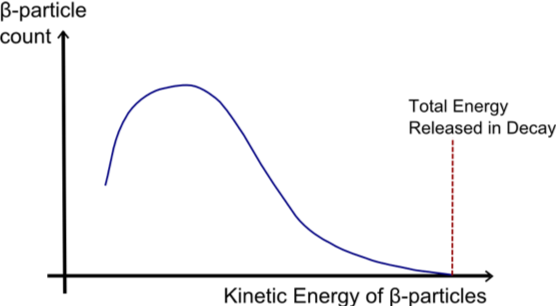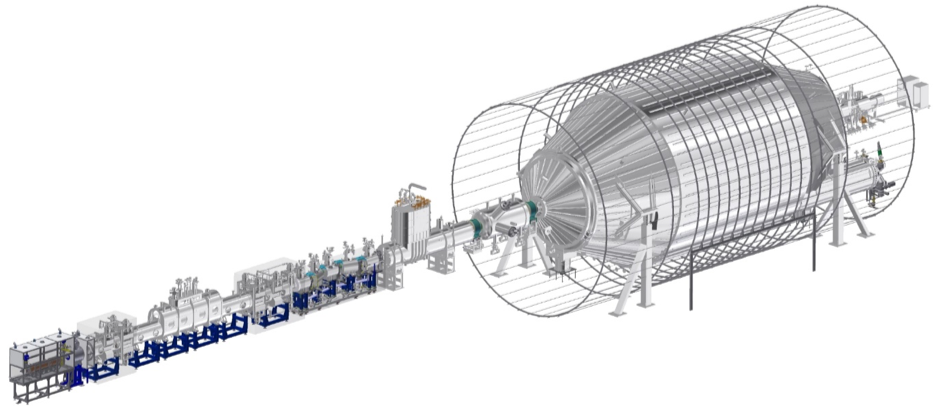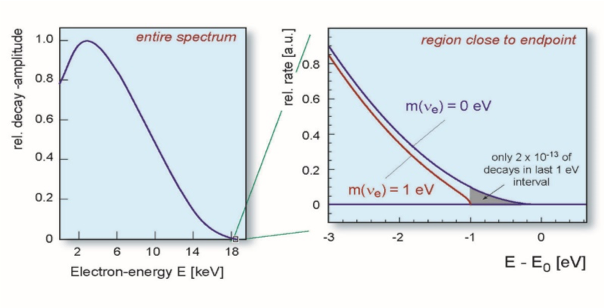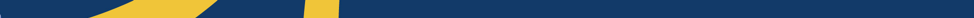# Letting the KATRIN out of the bag

By Benjamin Tam, February 12, 2020

Article: “Improved Upper Limit on the Neutrino Mass from a Direct Kinematic Method by KATRIN”
Authors: KATRIN Collaboration
Reference: https://journals.aps.org/prl/abstract/10.1103/PhysRevLett.123.221802

In my regular series, I will be talking about past, present, and future detectors used in particle astrophysics, and the impact these titanic monuments have had on our understanding of the universe. Today, we look at how even the simplest questions in the field require monumental endeavours.

When you need to know the value of basic properties of particles such as their masses and charges, it’s rare to need to look any further than Wikipedia. We take for granted the ease, accuracy and precision of these values. However, even the simplest measurements may require clever techniques: after all, you can’t just place an electron on a scale. This is a problem faced by neutrino scientists today.

The neutrino mass scale

Modern particle astrophysics stems from the observation that electron, muon and tau neutrinos – three fundamental Standard Model particles – can change from one type to another (see Flavour Oscillations). The implication of this discovery is that neutrinos do indeed have miniscule mass in contradiction to the Standard Model, the rulebook which particle physics has played by for over 50 years. This begs the question: what exactly is the mass of the neutrino? Atmospheric and solar neutrino experiments give us the difference in masses between the neutrinos, but the mass scale itself is unknown.Scientists attempting to measure miniscule mass of the neutrino. Source

The mass of the neutrino can be determined in three ways. First, neutrinos should have affected the formation of large-scale structures across the early universe, the degree of which is based on the neutrino mass. Second, certain hypothesized particle decays such as neutrinoless double beta decay limit the range of potential neutrino masses – observing these decays would narrow the search range. Both of these methods are strongly model dependent and therefore may never yield the desired measurement if the models do not end up properly representing the universe.

The third way to determine the mass scale of the neutrino is not only model-independent, but a direct measurement that can be performed. It relies on a precise investigation of the beta decay spectrum.

KAT and mouseAn illustration of a beta-decay energy spectrum.

Neutrinos have a special relationship with beta decay. It was due to beta decays that neutrinos were postulated in the first place. Traditionally, beta decay was empirically seen as a neutron decaying into a proton and electron (aka beta particle). As the neutron, proton and electron masses were fairly well known, the daughter products of this reaction should have had predictable energies due to the principle of conservation of energy. However, it was observed that the energy spectra (a histogram of beta particle energies from many decays) were continuous – an apparent violation of the conservation of energy. This discrepancy led to the discovery of a neutrino – a small, chargeless particle that does not interact through the usual forces.

The neutrino carries away some portion of the energy, leaving the kinetic energy of the beta particle as some value between the electron rest mass and the Q value (the total energy released in the decay). However, if the neutrino has mass, it means the high end-point of the energy spectrum –corresponding to a scenario where the neutrino has no kinetic energy – should be less than the Q-value. This is because the rest mass of the neutrino must also be taken into account: the greater the mass, the larger the difference between the Q-value and endpoint.A diagram of the KATRIN experiment. The cylinder to the right is the main spectrometer and is over 5 stories tall. (Credit: Karlsruhe Institute of Technology)

The name of the game is therefore a super-precise instrument able to measure exactly where the endpoint energy of the energy spectrum is.

Look what the KATRIN dragged in.

Because of a relatively low endpoint energy (E0 = 18.57 keV) and decent half-life (~12 years), the decay of tritium (3H –> 3He+ + e + νe) is a promising isotope that could be used to measure the discrepancy between the end-point and the Q-value. Several experiments have been attempting to measure tritium, but the undisputed current leader is the KArlsruhe TRItium Neutrino (KATRIN) Experiment.

KATRIN uses a gaseous molecular tritium source and attempts to measure the energy of the beta decays using spectrometer based on a technique called Magnetic Adiabatic Collimation with Electrostatic filtering (“MAC-E-filter”). A MAC-E-filter is effectively a pair of solenoids that magnetically distort the path of a beta particle based on its kinetic energy. The resulting “time-of-flight” (glorified travel time) of each beta particle can then be measured and the kinetic energy of the particle recovered. This technique allows for exceptional energy resolution with small systematics.

Not KATting aroundAn illustration of the latest KATRIN results, setting the constraint on the maximum neutrino mass to be 1.1 eV after 4 weeks of data. Source.

KATRIN recently released results from their first major campaign using high-purity tritium. From the measurements of the energy endpoint, the KATRIN collaboration managed to constrain the maximum mass of the neutrino to 1.1 eV (in comparison, the electron has a mass of 511,000 eV). This is a major improvement over the existing limit of 2 eV despite having only run for 4 weeks. After 1000 days of data taking, the KATRIN collaboration will be able to constraint the maximum mass to an incredible 0.2 eV.

Despite the immensity of the operation, KATRIN is trying to look at one of the most basic universal properties: the mass of a fundamental particle. The next time you google a simple constant, try to remember the enormous effort undertaken by generations of scientists it took to produce that for you.